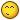## Recommended Posts

```do
local function exportstring( s )
return string.format("%q", s)
end
function table.save(  tbl,filename )
local charS,charE = "   ","n"
local file,err = io.open( filename, "wb" )
if err then return err end
local tables,lookup = { tbl },{ [tbl] = 1 }
file:write( "return {"..charE )

for idx,t in ipairs( tables ) do
file:write( "-- Table: {"..idx.."}"..charE )
file:write( "{"..charE )
local thandled = {}

for i,v in ipairs( t ) do
thandled[i] = true
local stype = type( v )
if stype == "table" then
if not lookup[v] then
table.insert( tables, v )
lookup[v] = #tables
end
file:write( charS.."{"..lookup[v].."},"..charE )
elseif stype == "string" then
file:write(  charS..exportstring( v )..","..charE )
elseif stype == "number" then
file:write(  charS..tostring( v )..","..charE )
end
end
for i,v in pairs( t ) do
if (not thandled[i]) then
local str = ""
local stype = type( i )
if stype == "table" then
if not lookup[i] then
table.insert( tables,i )
lookup[i] = #tables
end
str = charS.."[{"..lookup[i].."}]="
elseif stype == "string" then
str = charS.."["..exportstring( i ).."]="
elseif stype == "number" then
str = charS.."["..tostring( i ).."]="
end

if str ~= "" then
stype = type( v )
if stype == "table" then
if not lookup[v] then
table.insert( tables,v )
lookup[v] = #tables
end
file:write( str.."{"..lookup[v].."},"..charE )
elseif stype == "string" then
file:write( str..exportstring( v )..","..charE )
elseif stype == "number" then
file:write( str..tostring( v )..","..charE )
end
end
end
end
file:write( "},"..charE )
end
file:write( "}" )
file:close()
end
local ftables,err = loadfile( sfile )
if err then return _,err end
local tables = ftables()
for idx = 1,#tables do
for i,v in pairs( tables[idx] ) do
if type( v ) == "table" then
tables[idx][i] = tables[v]
end
if type( i ) == "table" and tables[i] then
end
end
for _,v in ipairs( tolinki ) do
tables[idx][v],tables[idx][v] =  tables[idx][v],nil
end
end
return tables
end
end```

pro ejemplo

```tabla={
minombre='Noa',
ano='23',
pais='Alemania'
}
table.save(tabla,"mitabla.lua")
for _,i in next, tabla do
say(i)
end
o
say("mi nombre es "..tabla.minombre.." tengo "..tabla.ano.." anos y bivo en "..tabla.pais)
```

##### Share on other sites

Gracias por el aporte pero seria mejor q pusieras informacion sobre lo q vas a postear!!!!!

solo DIGO NO!!!!

solo DIGO NO!!!!

que tu dieses ?

##### Share on other sites

Very good noa##### Share on other sites

Bueno cierro el tema a petición de autor, y borro mensajes de spam.